### Constructions -

CBSE Test Paper 01

CH-11 Constructions

1. With the help of a ruler and a compass, it is not possible to construct an angle of ____.
1. ${37.5}^{0}$
2. ${40}^{0}$
3. ${22.5}^{0}$
4. ${67.5}^{0}$
2. The construction of a triangle ABC, given that BC = 3 cm, $\mathrm{\angle }C={60}^{0}$ is possible when the difference of AB and AC is equal to ____.
1. 3.2 cm.
2. 3 cm.
3. 2.8 cm.
4. 3.1 cm.
3. In the adjoining figure, if $\mathrm{\angle }$2 = 55° and $\mathrm{\angle }$5 = 55°, the lines m and n are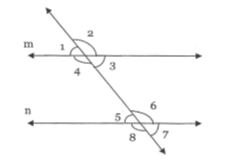1. cannot say
2. not parallel
3. parallel
4. none of these
4. With the help of a rular and a compass, it is not possible to construct an angle of _____.
1. ${67.5}^{0}$
2. ${7.5}^{0}$
3. ${35}^{0}$
4. ${82.5}^{0}$
5. Which of the following angles cannot be constructed with the help of a ruler and a compass?
1. $7{\frac{1}{2}}^{\circ }$
2. $37{\frac{1}{2}}^{\circ }$
3. $22{\frac{1}{2}}^{\circ }$
4. $30{\frac{1}{2}}^{\circ }$
6. Can we construct an angle of 67.5°? Justify for your answer

7. Construct an angle equal to a given angle.

8. Draw an obtuse angle. Bisect it. Measure each of the angles so obtained.

9. Using protractor, draw a right angle. Bisect it to get an angle of measure 45°.

10. Construct a triangle ABC with perimeter 12 cm, $\mathrm{\angle }$B = 60o and $\mathrm{\angle }$C = 45o.

11. Construct an angle of 90° at the initial point of a given ray and justify the construction.

12. Construct a triangle ABC with perimeter 10 cm and each base angle is of 45o.

13. Construct a right triangle ABC whose base BC is 6 cm and the sum of hypotenuse AC and other side AB is 10 cm.

14. Draw an angle of 110° with the help of a protractor and bisect it. Measure each angle.

15. Construct a triangle ABC in which BC = 7cm $\mathrm{\angle }$B = 75° and AB + AC = 9cm.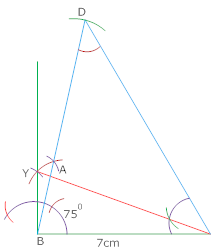CBSE Test Paper 01
CH-11 Constructions

Solution

1. (b) ${40}^{0}$
Explanation: With the help of a ruler and a compass, it is not possible to construct an angle which is not a multipe of ${15}^{0}$. Since ${40}^{0}$ ia not a multiple of ${15}^{0}$, so, we cannot construct it.
2. (c) 2.8 cm.
Explanation: The construction of $\mathrm{△}ABC$ is possible when difference of other two sides is less than its base i.e BC> AB-AC.
3. (b) not parallel
Explanation: For the parallel lines, the corresponding or alternate angles should be equal, but here $\mathrm{\angle }$2 and $\mathrm{\angle }$5 are neither corresponding nor alternate so, the lines m and n cannot be parallel.
4. (c) ${35}^{0}$
Explanation: With the help of a ruler and a compass, it is not possible to construct an angle which is not a multiple of ${15}^{0}$ and as in these option, ${35}^{0}$ is not a multiple of ${15}^{0}$ ,so we can not construct an angle of ${35}^{0}$.
5. (d) $30{\frac{1}{2}}^{\circ }$
Explanation: With the help of a ruler and a compass, we can not construct an angle which is not a multiple of ${15}^{0}$. Since $30{\frac{1}{2}}^{0}$ is not a multiple of ${15}^{0}$, so, we can not construct it.
6. Yes,  we can draw by constructing an angle of 135° with protractor and bisecting it with compass. (Since bisecting angles means dividing it into half, hence   $\frac{{135}^{\circ }}{2}$ =  67.5)

7. Given: $\mathrm{\angle }$POQ and a point A
Required: To construct an angle at A equal to $\mathrm{\angle }$POQ.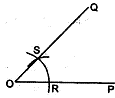Steps in construction:

1. With O as centre and suitable radius, draw an arc to meet OP at R and OQ at S.
2. Through A draw a line AB of any length.
3. Taking A as centre and radius equal to OR draw an arc to meet AB at D.
4. Measure the segment RS with compass.
5. With D as centre and radius equal to RS, draw an arc to meet the previous arc at E.
6. Join AE and produce it to C, then $\mathrm{\angle }$BAC is the required angle equal to $\mathrm{\angle }$POQ.
8. Steps of Construction:-

$\therefore$ $\mathrm{\angle }$ABR = $\mathrm{\angle }$RBC = 60o

1. Draw angle ABC of 120o.
2. With centre B and any radius, draw an arc which intersect AB at P and BC at Q.
3. With centre P and Q and radius more than $\frac{1}{2}$PQ, draw two arcs which intersect each other at R.
4. Join BR.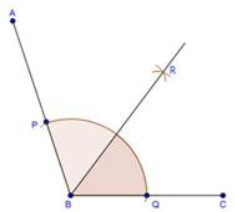9. Steps of Construction:-

$\therefore$ $\mathrm{\angle }$RBC = 45o.

1. Draw an angle ABC of 90o.
2. With centre B and any radius, draw an arc which intersects AB at P and BC at Q.
3. With centres P and Q and radius more than $\frac{1}{2}$PQ, draw two arcs which intersect each other at R.
4. Join RB.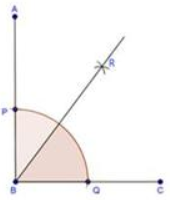10. Given: In triangle ABC, $\mathrm{\angle }$ B = 60o and $\mathrm{\angle }$C = 45and AB + BC + CA = 12 cm.
Required: To construct the triangle ABC.
Steps of construction :

ABC is the required triangle.

1. Draw a line segment XY = AB + BC + CA = 12 cm.
2. Make $\mathrm{\angle }$LXY = ∠B = 60o and $\mathrm{\angle }$MYX = $\mathrm{\angle }$C = 45o
3. Bisect $\mathrm{\angle }$LXY and $\mathrm{\angle }$MYX. Let these bisectors meet at a point A.
4. Draw the perpendicular bisectors PQ of AX and RS of AY.
5. Let PQ intersects XY at B and RS intersects XY at C.
6. Join AB and AC.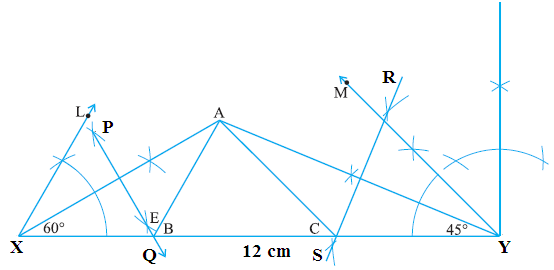11. Steps of construction:

1. Draw a ray OA.
2. With O as centre and convenient radius, draw an arc LM cutting OA at L.
3. Now with L as centre and radius OL, draw an arc cutting the arc LM at P.
4. Then taking P as centre and radius OL, draw an arc cutting arc PM at the point Q.
5. Join OP to draw the ray OB. Also join O and Q to draw the OC. We observe that: $\mathrm{\angle }$AOB = $\mathrm{\angle }$BOC = 60°
6. Now we have to bisect BOC. For this, with P as centre and radius greater than $\frac{1}{2}$ PQ draw an arc.
7. Now with Q as centre and the same radius as in step 6, draw another arc cutting the arc drawn in step 6 at R.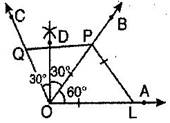8. Join O and R and draw ray OD.
Then $\mathrm{\angle }$AOD is the required angle of 90°.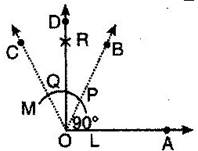Justification:
Join PL, then OL = OP = PL [by construction]
Therefore $\mathrm{\Delta }$OLP is an equilateral triangle and $\mathrm{\angle }$POL which is same as $\mathrm{\angle }$BOA is equal to 60°.
Now join QP, then OP = OQ = PQ [ by construction]
Therefore $\mathrm{\Delta }$OQP is an equilateral triangle.
$\therefore$ $\mathrm{\angle }$POQ which is same as $\mathrm{\angle }$BOC is equal to 60°.
By construction OD is bisector of $\mathrm{\angle }$BOC.
$\therefore$ DOC = $\mathrm{\angle }$DOB = $\frac{1}{2}\mathrm{\angle }$BOC = $\frac{1}{2}×$ 60° = 30°
Now, $\mathrm{\angle }$DOA = $\mathrm{\angle }$BOA + $\mathrm{\angle }$DOB $⇒$$\mathrm{\angle }$DOA = 60° + 30°
$⇒$ $\mathrm{\angle }$DOA = 90°
12. Given: In triangle ABC, $\mathrm{\angle }$B = 45o$\mathrm{\angle }$C = 45o and AB + BC + CA = 10 cm.
Required: To construct the triangle ABC.
Steps of construction :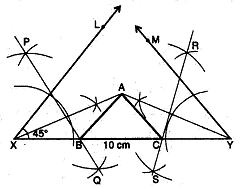ABC is the required triangle.

1. Draw a line segment XY = AB + BC + CA = 10 cm.
2. Construct $\mathrm{\angle }$LXY = $\mathrm{\angle }$B = 45o and $\mathrm{\angle }$MYX = ∠C = 45o
3. Bisect $\mathrm{\angle }$LXY and $\mathrm{\angle }$MYX. Let these bisectors meet at a point A.
4. Draw the perpendicular bisectors PQ of AX and RS of AY.
5. Let PQ intersect XY at B and RS intersect XY at C.
6. Join AB and AC.
13. Steps of construction:-

$\therefore$ $\mathrm{△}$ABC is the required triangle.

1. Draw a line segment BC of 6 cm.
2. At B, draw an angle XBC of 90o.
3. With centre B and radius 10 cm draw an arc which intersects XB at D.
4. Join DC.
5. Draw the perpendicular bisector of DC which intersects DB at A.
6. Join AC.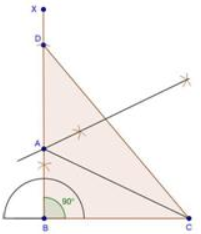14. Given: An angle ABC = 110°.
Required: To draw the bisector of $\mathrm{\angle }ABC$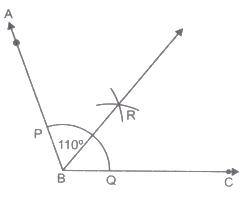Steps of construction:

1. With B as centre and a convenient radius draw an arc to intersect the ray’s BA and BC at P and Q respectively.
2. With centre P and a radius greater than half of PQ, draw an arc.
3. With Centre Q and the same radius (as in step 2), draw another arc to cut the previous arc at R.
4. Draw ray BR. This ray BR is the required bisectors of $\mathrm{\angle }ABC$.
15. Steps of construction:

1. Draw BC = 7cm
2. Draw $\mathrm{\angle }$DBC = 75°
3. Cut a line segment BD = 9cm
4. Join DC and make $\mathrm{\angle }$DCY =$\mathrm{\angle }$BDC
5. Let CY intersect BX at A
6. Triangle ABC is required triangle